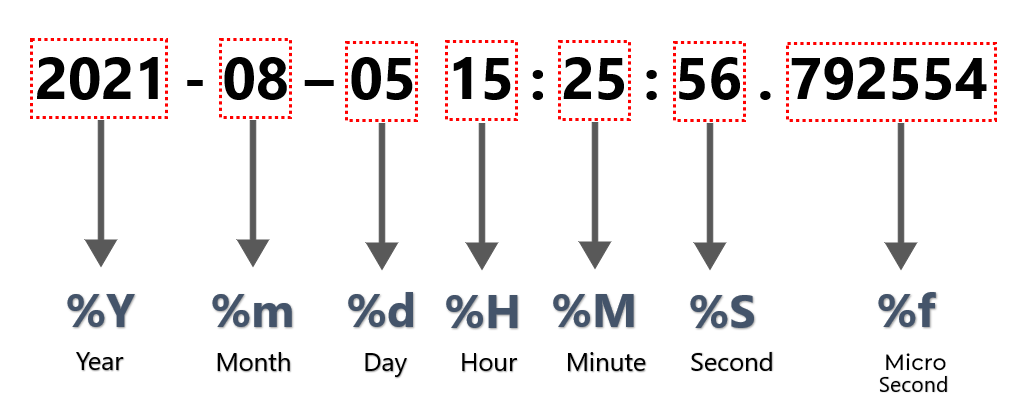Open in App
Not now

# Parsing DateTime strings containing nanoseconds in Python

• Last Updated : 09 Nov, 2022

Most of the applications require a precision of up to seconds but there are also some critical applications that require nanosecond precision, especially the ones which can perform extremely fast computations. It can help provide insights on certain factors related to time space for the application. Let us see how can we parse DateTime strings that have nanoseconds in them. Python has a list of directives that can be used in order to parse the strings as datetime objects. Let us have a look at some of them that we will be using in our codes.

#### Image Demonstration of DateTime object:Demonstration of DateTime object

Let us take the default Python timestamp format: “2021-08-05 15:25:56.792554” as an example to work on.

### Method 1: Using DateTime module

In this example, we will see that the nanoseconds value is 792554. “%f” directive is used to parse the nanoseconds. The same thing is cross-verified by converting the “%f” directive to the string value of its representation for the datetime object using the strftime() method.

## Python

 `from` `datetime ``import` `datetime` `# Parse the default python timestamp format``dt_obj ``=` `datetime.strptime(``"2021-08-05 15:25:56.792554"``,``                           ``"%Y-%m-%d %H:%M:%S.%f"``)` `# Verify the value for nano seconds``nano_secs ``=` `dt_obj.strftime(``"%f"``)` `# Print the value of nano seconds``print``(nano_secs)`

Output

`792554`

### Method 2: Using Pandas library

Here we will use pandas.to_datetime() methods to parsing DateTime strings containing nanoseconds.

Syntax:

pandas.to_datetime(arg, errors=’raise’, dayfirst=False, yearfirst=False, utc=None, box=True, format=None, exact=True, unit=None, infer_datetime_format=False, origin=’unix’, cache=False)

Parameters:

arg: An integer, string, float, list or dict object to convert in to Date time object.
dayfirst: Boolean value, places day first if True.
yearfirst: Boolean value, places year first if True.
utc: Boolean value, Returns time in UTC if True.
format: String input to tell position of day, month and year.

## Python

 `import` `pandas as pd` `# Parse the timestamp string by``# providing the format of timestamp string``dt_obj ``=` `pd.to_datetime(``"2021-08-05 15:25:56.792554"``,``                        ``format``=``"%Y-%m-%d %H:%M:%S.%f"``)` `# Verify the value for nano seconds``nano_secs ``=` `dt_obj.strftime(``"%f"``)` `# Print the value of nano seconds``print``(nano_secs)`

Output:

`792554`

The above example is similar to the earlier one except for the fact that we have used pandas library instead of the datetime module. This can prove to be handy when we are working with pandas dataframes. One beautiful thing about this library is that we might not need to provide the format manually. The parameter infer_datetime_format in the pandas.to_datetime() method can take care of that automatically if provided as True. In cases, it can increase the parsing speed by ~5-10x. Below is an example of the same.

## Python

 `import` `pandas as pd` `# Parse the timestamp string by``# providing infer_datetime_format as True``dt_obj ``=` `pd.to_datetime(``"2021-08-05 15:25:56.792554"``,``                        ``infer_datetime_format``=``True``)` `# Verify the value for nano seconds``nano_secs ``=` `dt_obj.strftime(``"%f"``)` `# Print the value of nano seconds``print``(nano_secs)`

Output:

`792554`

My Personal Notes arrow_drop_up